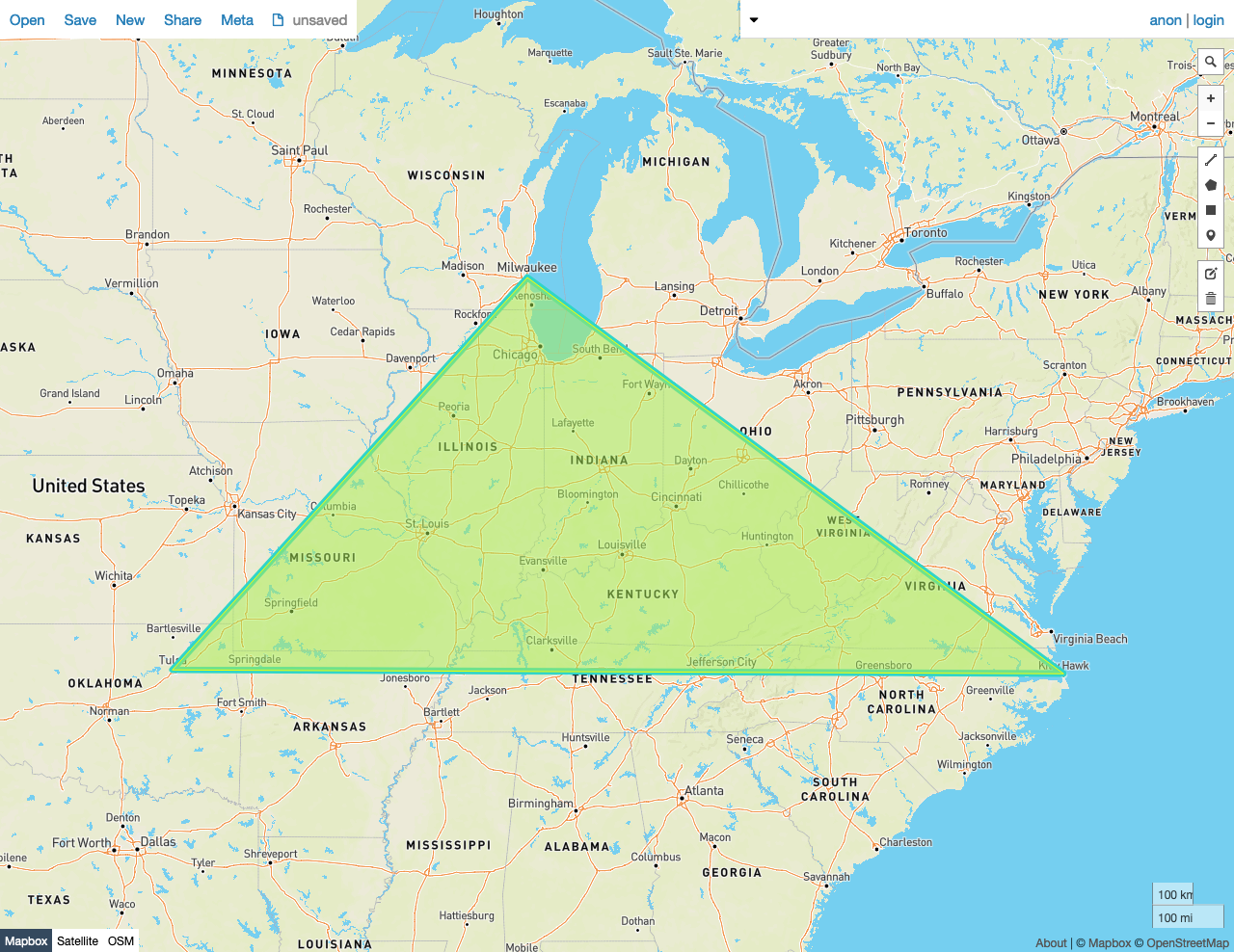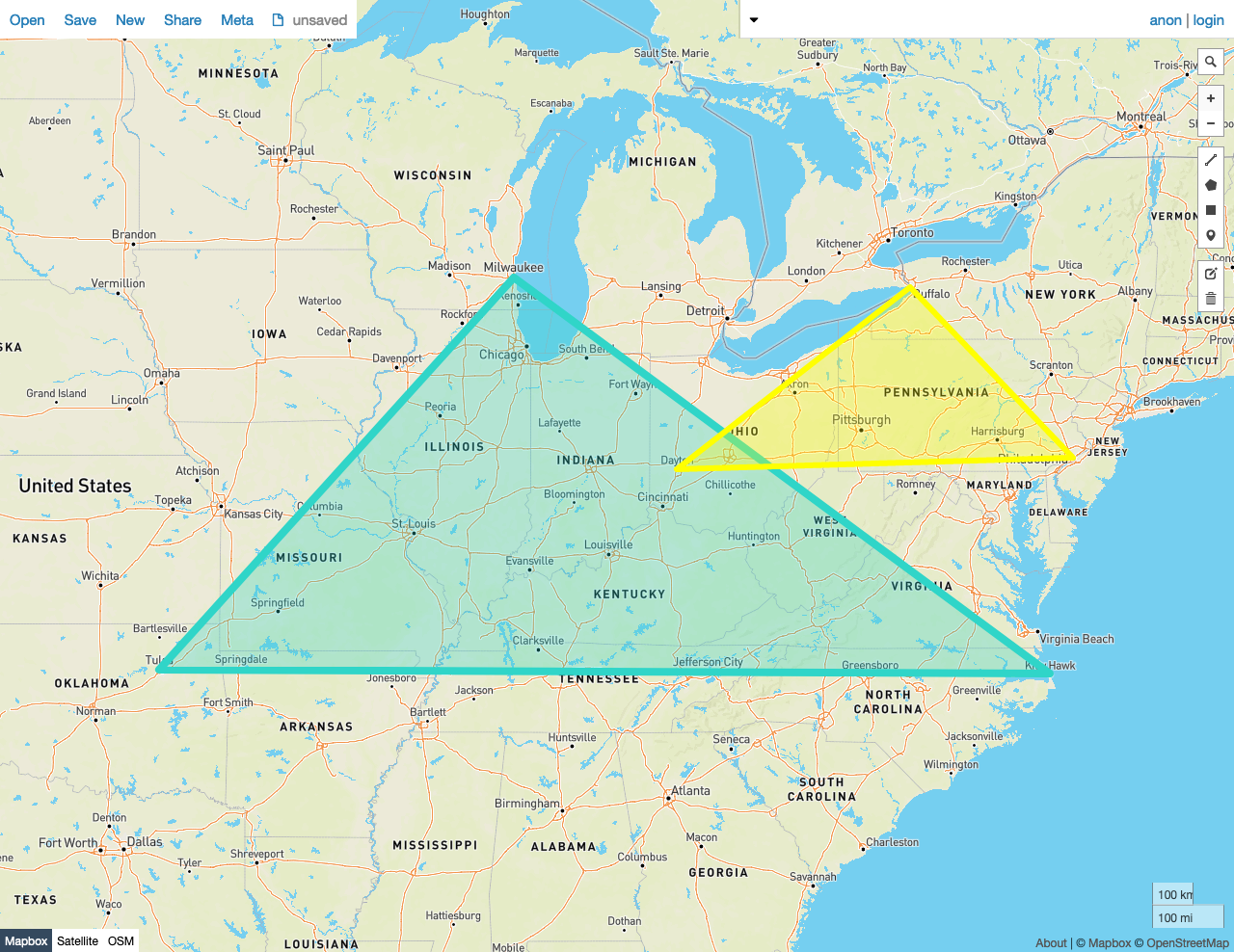On this pageGiven two shapes A and B, `ST_Equals(A, B)` returns `true` if every point in the set of points that make up A is also in B, and if every point in the set of points that make up B is also in A. The ordering of the points in A and B may differ, but they must be made up of equivalent sets.

Another way of describing `ST_Equals(A, B)` is that it will return `true` if both `ST_Within(A, B)` and `ST_Within(B, A)` also return `true`.

`ST_Equals` works on the following data types:

Note:

`ST_Equals` will attempt to use any available spatial index to speed up its operation. Use the prefixed variant `_ST_Equals` if you do not want any spatial indexes to be used.

## Examples

Note:

The screenshots in these examples were generated using geojson.io, but they are designed to showcase the shapes, not the map. Representing `GEOMETRY` data in GeoJSON can lead to unexpected results if using geometries with SRIDs other than 4326 (as shown below).

### True

In this example, `ST_Equals` returns `true` because:

• Every Point in the set of Points that make up Polygon A is also in Polygon B, and every Point in the set of Points that make up Polygon B is also in Polygon A

As mentioned above, the ordering of the points in A and B does not matter. Below, there are two triangles with the same vertices, which are ordered differently. In the screenshot, one triangle is drawn in yellow, and the other blue. The blue and yellow strokes of the two separate triangles' boundaries are visible. Because the images are overlaid on each other, the yellow and blue combine to make part of the exterior outline of the image a green color.

``````SELECT st_equals(st_geomfromtext('SRID=4326;POLYGON((-87.906471 43.038902, -95.992775 36.153980, -75.704722 36.076944, -87.906471 43.038902))'), st_geomfromtext('SRID=4326;POLYGON((-95.992775 36.153980, -87.906471 43.038902, -75.704722 36.076944, -95.992775 36.153980))'));
``````
``````   st_equals
---------------
true

(1 row)
``````### False

In this example, `ST_Equals` returns `false` because:

• It's obvious that not every Point that makes up the set of Points in Polygon A is not also in Polygon B.
``````SELECT st_equals(st_geomfromtext('SRID=4326;POLYGON((-87.906471 43.038902, -95.992775 36.153980, -75.704722 36.076944, -87.906471 43.038902))'), st_geomfromtext('SRID=4326;POLYGON((-84.191605 39.758949, -75.165222 39.952583, -78.878738 42.880230, -84.191605 39.758949))'));
``````
``````   st_equals
---------------
false
(1 row)
``````